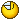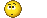# logical puzzle II

#### dfx

Registered
ok, here's one from me...

peter, simon and daniel are given the task to figure out two numbers. they get the following information: both numbers are between 1 and 1000 and whole numbers (no decimal numbers). peter is given the result of multiplying both numbers with each other (= the product), simon is given the sum of both numbers (= one number plus the other), and daniel is given the difference between both numbers (= one number minus the other).

however, they must not share those pieces of information with each other. but all three guys are mathematical geniuses, they can do any number of calculations in their head instantly.

then, the following conversation takes place:

peter: i don't know the numbers.
simon: you don't need to tell me that, because i knew that already.
peter: in that case, i know both numbers now.
simon: ok, i know them now too.
daniel: i still don't know the numbers. i can only guess a number, which is probably one of the numbers we're looking for, but i don't know for sure.
peter: i know which number you're talking about, but that one is wrong.
daniel: ok, then i also know both numbers now.​

which are the two numbers?

which are the two numbers?ok, here's one from me...

peter, simon and daniel are given the task to figure out two numbers. they get the following information: both numbers are between 1 and 1000 and whole numbers (no decimal numbers). peter is given the result of multiplying both numbers with each other (= the product), simon is given the sum of both numbers (= one number plus the other), and daniel is given the difference between both numbers (= one number minus the other).

however, they must not share those pieces of information with each other. but all three guys are mathematical geniuses, they can do any number of calculations in their head instantly.

then, the following conversation takes place:

peter: i don't know the numbers.
simon: you don't need to tell me that, because i knew that already.
peter: in that case, i know both numbers now.
simon: ok, i know them now too.
daniel: i still don't know the numbers. i can only guess a number, which is probably one of the numbers we're looking for, but i don't know for sure.
peter: i know which number you're talking about, but that one is wrong.
daniel: ok, then i also know both numbers now.​

which are the two numbers?

Got the first one but Faustus beat me to it! Determined to get this one in time now....good luck everyoneOK, I'm going out on a limb, are the numbers 2 and 6?

ehm, no. xDD

ehm, no. xDD
Damn you!*demands a truckload of headache medication from dfx4 and 13!If it's not I'm quitting4 and 13!If it's not I'm quittinghaha still no xDDDD

you gonna make half a million posts now, one for each combination?how you getting those numbers anyway?

*demands a truckload of headache medication from dfx1 and 999

1 and 999

nice try, but also no1 and 999

i can share my medication with youhaha still no xDDDD

you gonna make half a million posts now, one for each combination?how you getting those numbers anyway?
Well, so far as I can tell:

The product has to be divisible by more than just two numbers, which also means it can't be a prime number. I'm sure the sum must be even, which means both numbers must either be odd or both even, however, for Peter to reach his conclusion, the product has to be divisible only by either two odd or two even numbers, which in turn suggests that both of the numbers have to be quite low (otherwise there'd be more ways that they'd be divisible).

It's been 16 years since I took a maths class though so I'm a little rustysounds kinda good so far, even though i can't really confirm your statements, as i took quite a different route to solve thisso you may be right, but heck if i knowbut as a hint: you really need to go through the whole conversation to figure out the actual numbers. if you get a certain result without paying attention to all of the statements, it's probably not righti can share my medication with youthanks, i just want to see the result now, my brain has had enoughlast effort, 1 and 2

/me downs some pills

thanks, i just want to see the result now, my brain has had enoughthat might take a few days for someone to figure it outlast effort, 1 and 2

/me downs some pills

it's not that easy